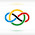### 2020 Peru EGMO TST #3

As I said in an earlier post, I will also post problems that are not from the IMO but I believe that they are of the same level.

Let $ABC$ be a triangle with $AB<AC$ and $I$ be your incenter. Let $M$ and $N$ be the midpoints of the sides $BC$ and $AC$, respectively. If the lines $AI$ and $IN$ are perpendicular, prove that the line $AI$ is tangent to the circumcircle of $\triangle IMC$.

Denote $X= IN\cap QC$. Let $F =(ABC)\cap AI$. Denote $Q$ as the reflection of $F$ over $O$, which is the center of $(ABC)$.

We know that

$$\measuredangle INM = \measuredangle INC- \measuredangle MNC$$
$$= \measuredangle QAC- \measuredangle BAC$$
$$= \measuredangle QBC- \measuredangle BQC$$
$$= \measuredangle QCB$$
$$= \measuredangle XCM$$
$$\implies MNXC\text{ is cyclic}.$$

Also, we can see that

$$\measuredangle CMX= \measuredangle CNX= \measuredangle CAQ$$
$$= \measuredangle CBQ$$
$$= \measuredangle QCB$$
$$= \measuredangle XCM$$
$$\implies MX=CX \implies X\text{ is the centre of } (CMQ).$$

Now because $$\measuredangle FIX=\measuredangle AIX=90^\circ=\measuredangle QCF=\measuredangle XCF$$, $FI=FC$, and triangles $XIF$ and $XCF$ share a side, we establish that $XI=XC$.

Therefore, $I$ is on $(CMQ)$ and then $FI^2=FC^2=FM\cdot FQ$ gives us that $AI$ is tangent to $(IMC)$, as desired. $\square$

1.Anonymous5/01/2022

by the way, what grade are you in?

2.Hi @anonymous!

3.Anonymous5/05/2022

oh nice nice
cool solution btw
i liked the problem as well. what is EGMO??

4.Anonymous5/05/2022

@above

I think @Imomath is referring to egmo.org (european girls math olympiad)

1.That's right.